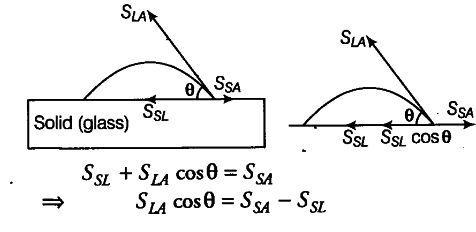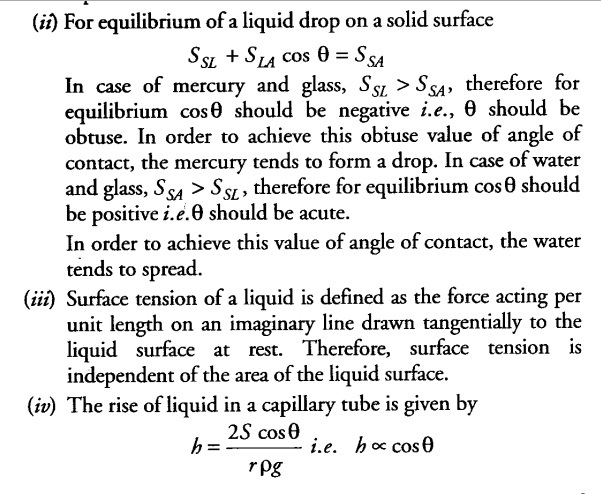# Explain why?The angle of contact of mercury with glass is obtuse

Explain why?
(i) The angle of contact of mercury with glass is obtuse, while that of water with glass is acute.
(ii) Water on a clean glass surface tends to spread out while mercury on the same surface tends to form drops. (Put differently, water wets the glass while mercury does not.)
(iii) Surface tension of a liquid is independent of the area of the surface.
(iv) Water with detergents dissolved in it should have small angles of contact.
(v) A drop of liquid under no external forces is always spherical in shape.

(i) When a small quantity of a liquid is poured on a sol’d, three types of interfaces namely liquid-air, air and solid-liquid are formed. The surface tension corresponding to these three interfaces are SLA, and SSL respectively. If θ is the angle of contact between solid and liquid then,Cos θ = Ssa - Ssl / Sla------(i)
For mercury and glass Ssa< SSL, therefore {torn Eq. (i) cos0 is negative and therefore 0 > 90° i.e., obtuse.
For water and glass Ssa, > SSL, therefore from Eq. (i) cos0 is positive and therefore0 < 90° i.e., acute.The cloth has narrow spaces in the form of fine capillaries. If angle of contact 0 is small, then the value of cos θ will be large and hence detergent will rise more in fine capillaries in the cloth. Now, the detergent solution will penetrate more in cloth and remove dust from the cloth.

(v)In the absence of external forces, the size of a liquid drop is decided only by the force due to surface tension. Due to surface tension, a liquid drop tends to acquire minimum surface area. As surface area is minimum for a sphere for a given volume of liquid. Therefore, under no external force a liquid is always spherical in shape.